# What is the PSD?

March 29, 2018

The power spectral density (PSD) estimates the distribution of a signal’s strength over a frequency spectrum. In vibration testing, the PSD graph is the most common tool for analyzing random vibration.

#### PSD Breakdown

Each word in “power spectral density” represents an essential component of the PSD.

Power: A signal’s mean-square value determines the PSD’s magnitude, meaning how far, on average, it strays from zero. For example, if a test engineer measures acceleration over time, then the mean of the squared acceleration values is the power of the PSD. In this case, “power” does not refer to a physical quantity, such as watts or horsepower.

Spectral: The PSD is a function of frequency. It represents the distribution of a signal over a spectrum of frequencies similar to a rainbow that represents the distribution of light over a spectrum of wavelengths (colors).

Density: The magnitude of the PSD is normalized to a single hertz (Hz) bandwidth. For example, for a signal with an acceleration measurement in unit G, the PSD units are G2/Hz.

The name power spectral density does not include the measured quantity, so engineers sometimes replace the word power with the name of the measurement. For example, they may refer to the PSD of an acceleration signal as the acceleration spectral density.

### Why Power?

The mean-square value (power) is a convenient measurement of signal strength. For example, Figure 2.1 displays the vibration time-history for a car’s floor panel measured by an accelerometer. The mean-square value equals 0.0053G2, and the root-mean-square (RMS) value equals 0.073G.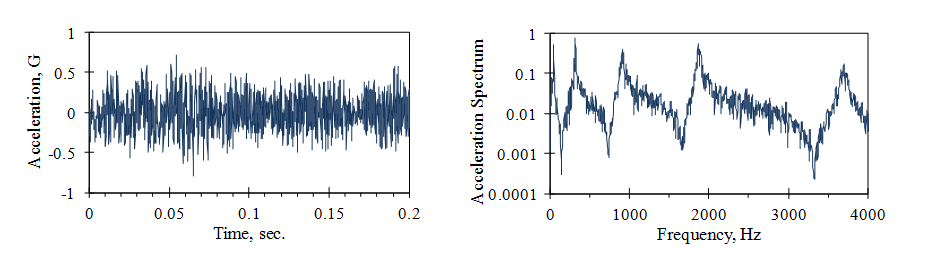Figure 2.1. The vibration of a car floor panel.

The signal’s average amplitude cannot be specified by the mean value because it is near zero. However, we can square the signal, which results in a positive quantity. Then, we can compute the mean-square value. To obtain a linear value (of acceleration, in this case), we can take the square root of the mean-square value to obtain the RMS.

The PSD plot can be created by an infinite number of waveforms, but when a PSD is defined, the gRMS will not change. This allows engineers to match a PSD to a specification and determine if the gRMS is as required. They can also use the gRMS to compare two PSD plots and verify that they are measuring the same amount of energy.

#### RMS Calculator Online

Automatically compute the root-mean-square (RMS) from a breakpoint table with the following random RMS calculator.

### Signals of Different Frequencies

We use the mean-square value to combine signals of different frequencies. For example, Figure 2.2 displays the summation of two sine waves with different frequencies. The mean-square value of the unit sine wave is 0.5, and the RMS value is 0.707. After the two waveforms are summed, the mean-square value is 1.0, and the RMS value is 1.0.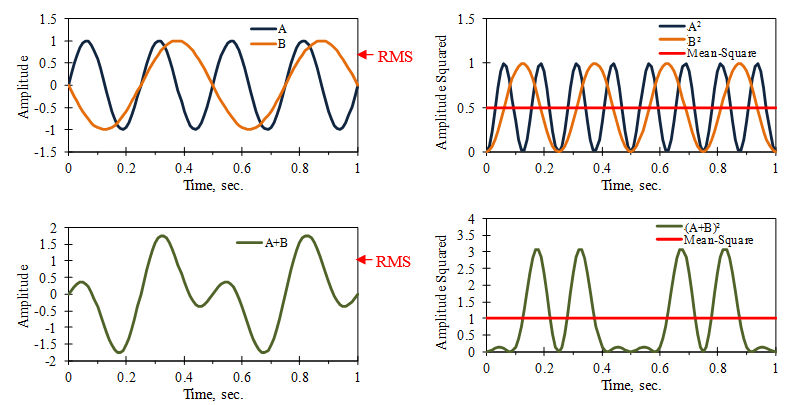Figure 2.2. The mean square value of A+B equals the sum of the mean-squares of A and B, where the mean values of A and B equal zero.

Mathematically, this is a general result for two independent variables A and B. The square of the sum is (A+B)2 = A2 + 2AB + B2.  If the two variables are independent and have a mean value of zero, then the mean value of 2AB is also zero (Figure 2.3).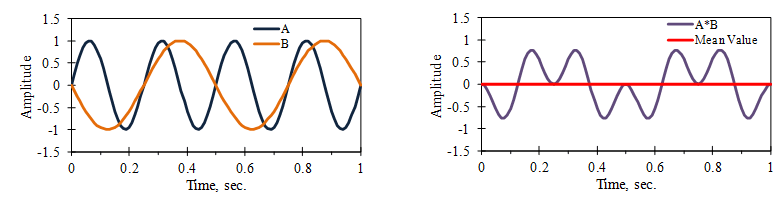Figure 2.3. The mean value of 2AB.

There are cases where the PSD is represented by the square root of its computed value. Therefore, an acceleration PSD may have units G/(Hz)1/2. Make sure to determine the PSD units.

### Why Spectral?

The frequency distribution of a signal provides useful information when dealing with systems that have resonances. In Figure 2.4, a cantilever beam was driven at the base by a broadband signal, which has a wide distribution of frequencies. An accelerometer measured the tip vibration.

It is difficult to determine the beam’s resonant frequency values from the signal’s time history (left). However, the peaks in the tip vibration’s frequency spectrum clearly show these values (right).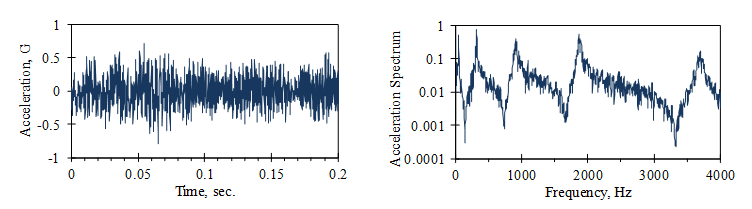Figure 2.4. Time history and frequency spectrum of beam vibration signals.

### Why Density?

The magnitude of a signal’s frequency distribution depends on the number of frequency bands in the distribution.

In Figure 2.5, the frequency spectrum of a car vibration signal is computed with three different frequency bandwidths. The squared magnitudes of the spectrum are proportional to the frequency bandwidth. To overcome this variation, the PSD divides the squared magnitude by the frequency bandwidth to provide a consistent value independent of the bandwidth.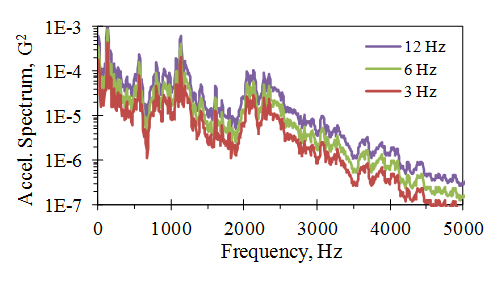Figure 2.5. Dependence of the frequency spectrum on the frequency bandwidth.

There are cases where individuals confuse the bandwidth-dependent frequency spectrum and the PSD. Make sure to determine if the bandwidth normalized the magnitude, which would be a feature of the PSD calculation.